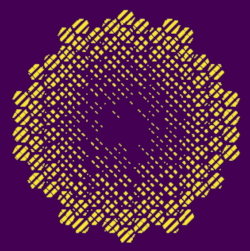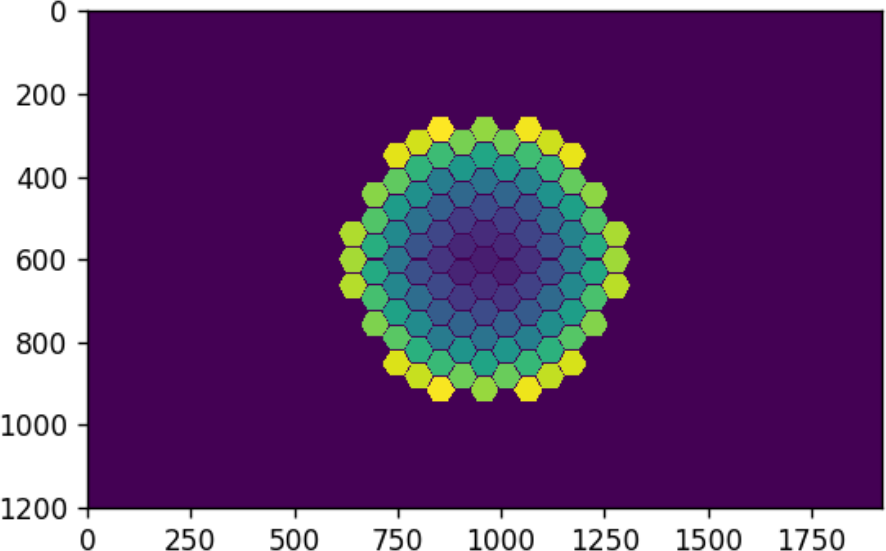# How to generate macropixel patterns for SLMs/DMDs with the Layout moduleIn many wavefront shaping experiments, such as for optimization experiments, like the seminal work by I. Vellkoop and A. Mosk, or for measuring the transmission matrix, one needs to control the amplitude and/or the phase of the field on a given number of macropixels (i.e. groups of pixels). Using DMDs, amplitude, and phase modulation can be achieved using the Lee hologram method and then sending the binary images to the device using the for ALP4lib in Python for Vialux DMDs. I released here a module written by M. W. Matthès and myself to easily and efficiently generate such patterns. The code can be found on my Github account here as well as an amplitude and phase modulation example: layout_amplitude_phase_modulation.ipynb.

### Creation of the layout

Let's create a layout to fit the size of the DMD that we control using ALP4lib.

import numpy as np
import matplotlib.pyplot as plt
from ALP4 import *
import Layout
DMD = ALP4(version = '4.3', libDir = 'C:/Program Files/ALP-4.3/ALP-4.3 API')
# Initialize the device
DMD.Initialize()
# Get the resolution of the DMD
dmd_res = [DMD.nSizeY,DMD.nSizeX]
center = [DMD.nSizeY//2,DMD.nSizeX//2]
# Creates an hexaonal Layout
hexSize = 20,
resolution = dmd_res,
center = center,
gap = 3)
# Retrieve the number of segments
npix = layout.nParts
# Display the layout pattern
layout.showLayout()

The created layout has the aspect shown in Fig 1. Each hexagon can be controlled independently.Figure 1. Hexagonal layout.

### Integration with ALP4lib

We want to send a sequence of 10 images on the DMD to modulate randomly the phase of the segments. For a more in-depth example about both amplitude and phase modulation, see my example layout_amplitude_phase_modulation.ipynb. We use here a period of the Lee hologram of 8 pixels and an angle for the grating of 45 degrees. We will use bitplanes instead of 8-bit images. That allows sending 8 times smaller arrays for faster loading (please read the ALP Vialux documentation of more information about bitplanes).

nbImg = 10
DMD.SeqAlloc(nbImg = nbImg, bitDepth = 1)
# Very important, we tell the DMD here that we will use bitplanes
DMD.SeqControl(ALP_DATA_FORMAT,ALP_DATA_BINARY_TOPDOWN)
for ind in range(nbImg):
# Generate a random phase vector of the same size as the number of macropixels.
vec = np.exp(1j*np.random.rand(npix)*2.*np.pi)
# Convert to bitplane
bitPlane = layout.getBitPlaneFromVec(vec, leePeriod=8, angle = np.pi/4, dataFormat = 'C')
# Send data to the DMD
DMD.SeqPut(imgData = bitPlane, PicOffset = ind, PicLoad = 1, dataFormat = 'C')

# Set image rate to 50 Hz
DMD.SetTiming(pictureTime = 20000)
# Run the sequence once
DMD.Run(loop = False)

Bitplanes are not directly viewable, the basic idea is that each byte corresponds to the bit values of 8 consecutive pixels. To take a look at the corresponding amplitude mask, we can use:

mask = layout.getMaskFromBitPlane(bitPlane)
plt.figure()
plt.imshow(mask)

The resulting amplitude mask is shown in Fig 2.

Figure 2. Binary mask corresponding to a random phase in vector.

The Layout module currently supports hexagonal, square, and diamond shape cells with the same syntax. Simply replace Hexagons() by Diamonds() or Squares().

### Citing this work

If you find this tool useful, please consider citing our paper: M. Matthès, P. del Hougne, J. de Rosny, G. Lerosey, and S. Popoff, "Optical complex media as universal reconfigurable linear operators," Optica 6, 465-472 (2019).

Created by sebastien.popoff on 21/10/2019